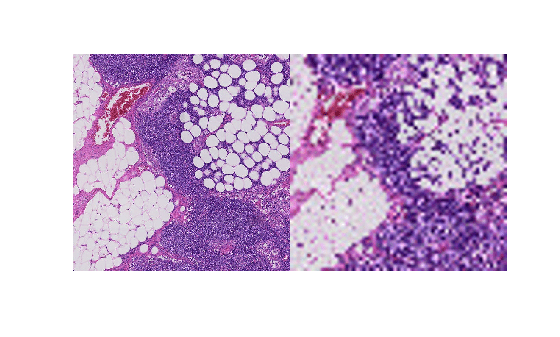# getRegion

Read arbitrary region of blocked image

## Syntax

``pixels = getRegion(bim,pixelStart,pixelEnd)``
``pixels = getRegion(bim,pixelStart,pixelEnd,'Level',L)``

## Description

example

````pixels = getRegion(bim,pixelStart,pixelEnd)` returns all the pixels in the blocked image `bim`, in the region specified by `pixelStart` and `pixelEnd`. ```
````pixels = getRegion(bim,pixelStart,pixelEnd,'Level',L)` gets the specified region from the `L`'th resolution level of the multiresolution `blockedImage`, `bim`. `pixelStart` and `pixelEnd` are pixel subscripts at the `L`'th resolution level.```

## Examples

collapse all

Create a blocked image.

`bim = blockedImage('tumor_091R.tif');`

Specify a region in the blocked image and retrieve the data.

```pstart_l1 = [2100, 1800, 1]; pend_l1 = [2600, 2300, 3]; imL1 = getRegion(bim, pstart_l1, pend_l1, "Level", 1);```

Convert the start and end points of the region to world coordinates.

```wstart_l1 = sub2world(bim, pstart_l1, "Level", 1); wend_l1 = sub2world(bim, pend_l1, "Level", 1);```

Convert the world coordinates to level 3 pixel subscripts.

```pstart_l3 = world2sub(bim, wstart_l1, "level", 3); pend_l3 = world2sub(bim, wend_l1, "level", 3); imL3 = getRegion(bim, pstart_l3, pend_l3, "Level", 3);```

Display the region in both resolution levels.

`montage({imL1, imL3});`## Input Arguments

collapse all

Blocked image, specified as a `blockedImage` object.

Starting pixel of the region, specified as a 1-by-N vector of pixel subscripts in the form `[row,column,...,subN]`, where N is the dimensionality of blocked image `bim`. Specify the pixel subscripts at the desired resolution level.

Ending pixel of the region, specified as a 1-by-N vector of pixel subscripts in the form `[row,column,...,subN]`, where N is the dimensionality of blocked image `bim`. Specify the pixel subscripts at the desired resolution level.

Resolution level, specified as a positive integer that is less than or equal to the number of resolution levels of `bim`.

## Output Arguments

collapse all

Pixels in specified region, returned as a numeric array.